# Adding Fractions: The Common Error

This is one of the most common mistakes students make when adding fractions:

For a long time, I thought that students did this solely based on confusion with multiplication. While we teach addition of integers before multiplication, addition of fractions is a more complicated process.

To multiply fractions, multiply the numerators and multiply the denominators. That’s it.

As far as a mathematician is generally concerned, this is fine. Mathematics teachers, who are a related but different species, will tend to want students to simplify.

Usually, as a high school teacher, I want students to show an understanding of the multiplication process (especially when it comes to, say, multiplying rational functions), so I separate the two steps of “multiplying” and “simplifying”, but in the interest of not upsetting the elementary school teachers who might be reading this, we should also simplify:

To recap: To multiply two numbers written in (proper or improper) fractional form, multiply the numerators and multiply the denominators. If needed, desired, or expected, “simplify” by factoring out any common factors. (Don’t say “cancel” or half the mathematics teachers on the internet will scream at you.)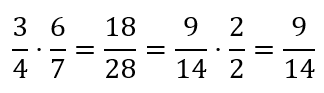2/2 = 1, so we don’t need to write it.

Division of fractions is more complicated, especially when there’s nesting going on, and it can get very confusing. Even more so when teachers insist on finding real world models and going for conceptual understanding instead of just letting students KFC (fried chicken, yum!).

That’s a separate rant, because I want to get back to addition, but I’m me, so here’s a sidebar.

Many teachers just teach an algorithm: To divide fractions, multiply by the reciprocal. But to keep away from the fancy schmancy (rigorous, correct) vocabulary, they teach “keep-change-flip”: That is, keep the first fraction the same, change the sign to multiplication, and then flip the second fraction: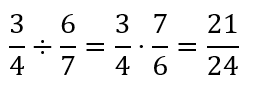Keep-Change-Flip, or “Division is multiplication of the reciprocal”

At some point the Keeper of the Acronyms noticed that KCF was painfully close to a common fast food place name, so they kept-changed-flipped the acronym itself to KFC, “Keep the first, Flip the second, Change the sign.”

Understandably, the other end of mathematics teachers then screamed about pointless algorithms and vapid acronyms and … good so far, but then … started insisting the teaching division of fractions had to be rooted in deep conceptual understanding of the process of division, and had to be siloed away from multiplication as much as possible.

This births questions like, “Jimmy has five and three-fours dollars and a pound of peas costs one and two-thirds dollars. How many pounds of peas can Jimmy buy?”

Which, okay, when you put it that way, isn’t a hellaciously pointless thing to do, but still. At some point, I think we need to stop insisting that every mathematical expression reflect a real-world scenario and that there are times (helllllooooo calculus!) when we’re just pushing numbers and letters around for practice.

Besides, I think this encapsulates a perfectly fundamental understanding of division in general:

Mathematically, division is equivalent to multiplying by the reciprocal.

However we get there, grand. KFC is painfully close to that, but it’s been covered in batter and fried in cuteness.

End of sidebar.

So, back to addition (and by implication, subtraction).

Multiplication, not so difficult. Division, one more step (replace the divisor by its reciprocal, and then multiply). Addition?

We cannot add fractions with different denominators. A Common Core aficionado will tell you that, conceptually, this means we’re not adding fractions at all. What we’re doing is rewriting to the same units, then adding the quantities of those units. That is: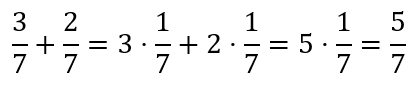Because 3 apples and 2 apples make 5 apples.

In the middle two expressions of the diagram, 1/7 is not a numeric value, it’s the unit “1/7 of the whole”, whatever “the whole” represents. So if the whole is a week, 3/7 + 2/7 means “three days plus two days”.

But if we want to skip concepts (or shortcut once we’ve explained), we can say “make the denominators the same, then add the numerators and leave the denominator alone.”

Even so, there are cases where competing efficiencies lead to different strategies. For instance, the easiest thing to teach is: When the denominators are different, multiply each fraction by a version of 1 equivalent to the other denominator divided by itself.

Um, what?

This:

This method always works, but there are times when it’s less efficient. It works best when the denominators are co-prime (or relatively prime, pick your terminological poison). Go explain “co-prime” to the average (checks Common Core State Standards) fifth grader. I’ll wait.

That’s not to say that a fifth grader couldn’t understand the notion of being co-prime, but the word “prime” doesn’t even appear in the Common Core State Standards. Not once.

Okay, it’s another rant that the key word for something called the Fundamental Theorem of Arithmetic doesn’t appear anywhere in the CCSS. Another day.

Part of that same rant:

Anyway, this method of adding fractions requires another step when the denominators have a common factor, and can often lead to larger numbers than younger students are comfortable around.

Taking a simple case, for instance: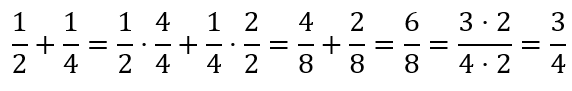It works, but, as the kids say, “you’re doing too much”.

Using models, it’s fairly easy to see that 1/2 + 1/4 = 3/4. It’s also fairly easy to see that 1/2 = 2/4. If we want to stick with models, it doesn’t make sense to convert these fractions to eighths.

Teachers often teach a concept of “lowest common multiple”, something which makes perfect sense to a mathematicians well-versed on the Fundamental Theorem of Arithmetic, which says some version of:

“All integers greater than one can be expressed uniquely as a product of powers of primes.”

But if you’re dealing with math standards that don’t include words like “arithmetic” or “prime” (I’m looking at you, CCSS!), that’s a bit trickier.

To students who are still struggling with the basics of number theory, then, the concept of “lowest common multiple” sits in Yet Another Algorithm: List a bunch of multiples of each number, then look for the first number that’s the same.

For 2 and 4, no big deal: 2’s multiples are 2, 4… there we go, done.

If I, the possessor of a Bachelor degree in Mathematics and Computer Science, wish to find the lowest common multiple, I use my understanding of the aforementioned Fundamental Theorem of Arithmetic: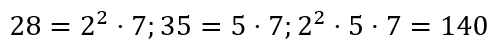Okay, that wasn’t so hard, was it?

But what students are often taught to do instead is:

28, 56, … hold on … 74, … can I use the calculator? … 102, 130, 158…

Oops, I made a mistake, but since I’m not allowed to use the calculator, I don’t know it. Now I hate math. Congratulations, Ms. Crabapple.

Or we could just go ahead and do what I suggested at first by multiplying each fraction by a form of one using the other fraction’s denominator.

But again, Ms. Crabapple is standing over us and side-eyeing us when we touch the calculator: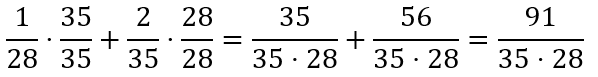I’m not multiplying that denominator without a calculator. Forget it.

You know what, screw this noise, I’m just going to do the same thing we did with multiplication: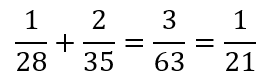This is not correct. Except… maybe sometimes?

I used to think this was the entire reason students did this: The addition rule is far too complicated, the multiplication rule is easy, just apply the easier rule.

And while I think this might be why some students do this, there’s another reason. Indeed, today I used the exact scenario where… you may want to sit down for this… this makes sense.

I was writing up a report on what portion of my students had reported in from the COVID-19 quarantine. I listed each class, then I provided a total.

I’ll make up some numbers because FERPA:

• First hour: 6/27
• Second hour: 12/25
• Third hour: 8/26
• Total: 26/78 = 1/3

There is nothing mathematically flawed about the bullets above. Each hour has a certain ratio of respondents. To get the total, I added up the values for the three classes… except I did NOT do this: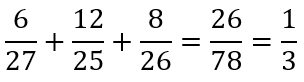This is not correct. But why not?

Okay, so, explain to a student why this is wrong.

Each class has an associated fraction. Check.

We want to know the combined fraction. Check.

“Combined” is accomplished through adding. Check.

So we want to add the fractions… right? WRONG!

The reality is: Students who do this are performing a valid mathematical operation of some sort. It involves adding. It involves fractions. It’s just not “addition of fractions”.

The misunderstanding goes back to a fundamental misunderstanding of what fractions represent, and what “addition of fractions” represents. Rather than throwing our hands up in frustration and repeating the same explanation yet again, we teachers need to take a step back and re-examine what a fraction means in the first place.

There are two study groups in afterschool tutoring. One of them has two students, a boy and a girl. The other has four students, one of whom is a girl. What portion of the entire afterschool tutoring group are girls?

This is a perfectly valid mathematical story problem, it’s something that you may well want to ask students at some point, in some version. There’s nothing weird about this at all. Also: It involves fractions. It involves adding. It does not involve adding fractions. Indeed, I can’t think of a way to write a problem that involves adding fractions that gives us the desired result of 2/6 (that is, 1/3).

We could define a different operator, such that the result is the sum of the numerators divided by the sum of the denominators, possibly simplified.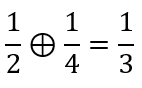Now this is fine, with our definition.

We could then explore the properties of this operator. Its inverse isn’t useful:

… but this is because of a fundamental flaw in our reasoning. We can’t simplify without losing key information.

If we instead defined our operator to disallow simplification, there’s no problem. Our inverse works fine.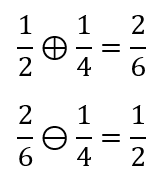Cool cool cool. (If you didn’t say that in a Jake Peralta voice, I’m disappointed.)

This is because, with our new operator, a “whole” is whatever’s represented by each integer. One whole student out of a group of two whole students combined with one whole student out of a group of four whole students makes two whole students out of a group of six whole students. 2/6 = 1/3 is not a valid conversion if we want to retain how many students we started out with.

Which gets to another important concept that we mathematics teachers often just gloss over: 1/3 is not identical to 2/6. The numeric value represented by 1/3 is equal to the numeric value represented by 2/6. Numerically, they’re identical; contextually, they may not be.

In probability-based exercises, we may prefer to stick to 52nds even if there are simpler forms of the fractions because we’re dealing with poker decks and we want to reinforce that. 13/52 is numerically equivalent to 1/4, but only the first one openly reminds us of our context.

The addition of fractions, meanwhile, involves assuming that the “whole” in the context is whatever it is we have a fraction of.

This is a harder concept than “each number represents a count of something”. And we usually teach fractions primarily in terms of “each number represents a count of something”… and then get annoyed when students take us at our word by doing:

Now, before you say, “Wait, I teach fifth grade, and I most certainly do NOT teach fractions like that!”

Based on the materials I’ve seen, yes, most curricula teach fractions exactly that way.

When you teach that “1/5 represents one piece of a star broken into five pieces”… that’s the most prominent message.

A while ago, there was a meme of an alleged question, answer, and teacher scold along these lines:

Q. Jimmy ate half a pizza. Dan ate a third of a pizza, but Dan ate more pizza than Jimmy. How is that possible?

Student answer: Dan’s pizza was larger.

Teacher response: No, it is impossible. 1/3 is less than 1/2.

I don’t know if this was a real problem or if this was made up to show how smart students are and how stupid teachers are. That’s not the point.

The point is, the item shows the very real confusion between what fractions are allowed to represent, under what conditions we can equate fractions, and what the standard operators on fractions work on.

It only makes sense to add and subtract when the fractions represent portions of comparable wholes. That is, when our underlying base unit is whatever the fraction is a fraction of, not when our underlying base unit is whatever our individual numbers represent the counts of.

This is not a simple concept. This is not an intuitive concept. If our students don’t understand how to add fractions, this may be why.

Which raises the question: Why doesn’t this create a problem for multiplication and division? Isn’t multiplication just repeated addition?

If you haven’t already, read Keith Devlin’s wonderful rant, “It Ain’t No Repeated Addition.” In it, he points out that multiplication of fractions is often the first time this argument seriously breaks down:

“And telling them that multiplication is repeated addition definitely requires undoing later.

How much later? As soon as the child progresses from whole-number multiplication to multiplication by fractions.”

When we add fractions, it works best conceptually if we think of denominators as units. Note that does not mean the underlying unit is the denominator. Rather, whatever we define as a “whole” (be it a real or abstract object), our fractional units are equally sized pieces of that whole.

When we multiply fractions, it doesn’t matter whether we conceptually think of denominators as units or as numeric values. Why not?

Adding things means we add things of the same unit. The unit must be the same. Seven bananas plus five bunches of bananas is not 12 in any sense… we need to know how many bananas are in each bunch.

Multiply things means we multiply the numbers AND the units. Consider 3/4 times 1/2. We could:

• Divide our “whole” into eight pieces. Take 3 · 1 = 3 of those pieces, for 3/8.
• Write our fractions as multipliers and units: 3 · 1/4 · 1 · 1/2, then multiply the numbers (3 · 1 = 3) and the units (1/4 · 1/2 = 1/8), then recombine (3/8).

Mathematically, these are the same process because multiplication multiplies both numeric values and units. Conceptually, they’re different, but it doesn’t really matter how students understand them when multiplying fractions. Either understanding will give them the same result.

Notice that while the issue with multiplying units might not be an issue with fractions, it is an issue elsewhere, such as geometry.

{A, B, C} are collinear points, with B between A and C. If |AB|= 3 cm and |BC|= 5 cm, how long is |AC|? To answer this, students need to make sure the units are the same, then add the numeric values: |AC|= 8 cm.

ABCD is a rectangle. If |AB| = 3 cm and |BC| = 5 cm, find the area and perimeter of ABCD. The perimeter is the sum of the four segments, so 16 cm… same unit. The area is the product: 15 cm²… new unit.

Because we often skip units in geometry classes, I’ve heard students confused with this:

ABCD is a square with side length 1. Find the area and perimeter of ABCD. The perimeter is 4 and the area is 1… how can the perimeter be greater than the area?

The answer is that we’re comparing two different units, but because we don’t write them, it’s confusing.

The point being, multiplication and addition are fundamentally different operators. Each causes confusion in its own way.

It’s easy for those of us who know how to perform the basic operations on fractions to see the underlying processes are simple. As teachers, we want to try to see these topics from the perspective of students who don’t understand.Clio Corvid